Class 10 RD Sharma Solutions – Chapter 15 Areas Related to Circles – Exercise 15.4 | Set 2

• Last Updated : 03 May, 2021

Question 18. In the given figure, Find the area of the shaded region. (Use π = 3.14).

Solution: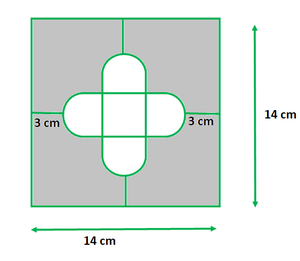The side of the square = 14 cm

So, area = side2

142 = 196 cm2

Let’s assume the radius of each semi-circle be r cm.

Then,

r + 2r + r = 14 – 3 – 3

4r = 8

r = 2

So, the radius of each semi-circle is 2 cm.

Area of 4 semi-circles = (4 x 1/2 x 3.14 x 2 x 2) = 25.12 cm2

Now,

the length of side of the smaller square = 2r = 2 x 2 = 4 cm

So, the area of smaller square = 4×4 = 16 cm2

Now we find the area of unshaded region = Area of 4 semi-circles + Area of smaller square

= (25.12 + 16) = 41.12 cm2

Now we find the area of shaded region = Area of square ABCD – Area of unshaded region

= (196 – 41.12) = 154.88 cm2

Hence, the area of the shaded region 154.88 cm2

Question 19. In the Figure, OACB is a quadrant of a circle with centre O and radius 3.5 cm. If OD = 2 cm, find the area of the

Solution: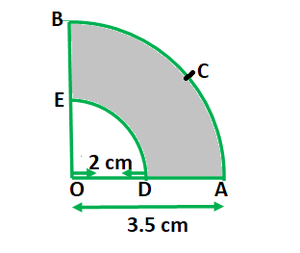Given that,

(i) Area of quadrant OACB = 1/4 πR2

= 1/4 (22/7)(3.5)2

= 269.5/28 = 9.625 cm2

= 1/4 π(R2 – r2)

= 1/4 (22/7)(3.52 – 22)

= 1/4 (22/7)(12.25 – 4)

= 1/4 (22/7)(8.25)

= 6.482 cm2

Hence, the area of the quadrant OACB is 9.625 cm2 and shaded region is 6.482 cm2

Question 20. In the figure, a square OABC is inscribed in a quadrant OPBQ of a circle. If OA = 21 cm, find the area of the shaded region.

Solution: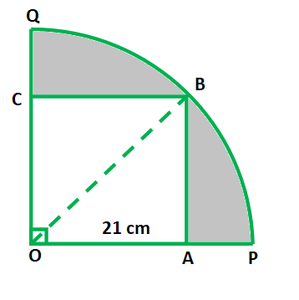Given,

Side of the square = 21 cm = OA

Area of the square = OA2 = 212 = 441 cm2

Diagonal of the square OB = √2 OA = 21√2 cm

From the figure its seen that,

The diagonal of the square is equal to the radius of the circle, r = 21√2 cm

So,

The area of the quadrant = 1/4 πr2 = 1/4 (22/7)(21√2)2 = 693 cm2

Now we find the area of the shaded region = Area of the quadrant – Area of the square

= 693 – 441

= 252 cm2

Hence, the area of the shaded region is 252 cm2

Question 21. In the figure, OABC is a square of side 7 cm. If OAPC is a quadrant of a cirice with centre O, then find the area of the shaded region. (Use π = 22/7)

Solution: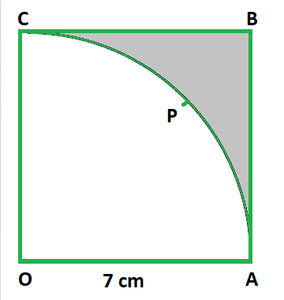Given that,

Side of OABC square = 7 cm

So, OA = AB = BC = OC = 7 cm

Now, the area of square OABC = side2 = 72 = 49 cm2

It is given that OAPC is a quadrant of a circle with centre O.

So, the radius of the quadrant = OA = OC = 7 cm

Area of the OAPC quadrant = 90/360 x πr2

= 1/4 x (22/7) x 72

= 77/2 = 38.5 cm2

Now we find the area of shaded portion = Area of square OABC – Area of quadrant OAPC

= (49 – 38.5) = 10.5 cm2

Hence, the area of shaded portion is 10.5 cm2

Question 22. In the figure, OE = 20 cm. In sector OSFT, square OEFG is inscribed. Find the area of the shaded region.

Solution: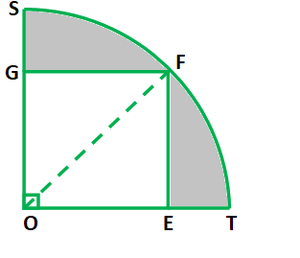Given that,

Side of OEFG square = 20 cm.

So its diagonal = √2 side = 20√2 cm

r = 20√2 cm

Now we find the area of the shaded portion = Area of quadrant – Area of square

= 1/4 πr2 – side2

= 1/4 (22/7)(20√2)2 – (20)2

= 1/4 (22/7)(800) – 400

= 400 x 4/7 = 1600/7 = 228.5 cm2

Hence, the area of the shaded region 228.5 cm

Question 23. Find the area of the shaded region in the figure, if AC = 24 cm, BC = 10 cm and O is the centre of the circle. (Use π = 3.14)

Solution: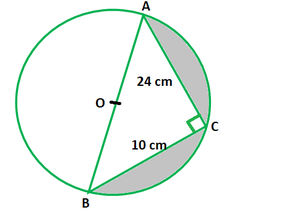Given,

AC = 24 cm and BC = 10 cm

From the figure AB is the diameter of the circle

So, ∠ACB = 90o

By using Pythagoras theorem

AB2 = AC2 + BC2 = 242 + 102 = 576 + 100 = 676

So, AB = √676 = 26 cm

So, the radius of the circle(r) = 26/2 = 13 cm

Now we find the area of shaded region = Area of semi-circle – Area of triangle ACB

= 1/2 πr2 – 1/2 x b x h

= 1/2 (22/7)132 – 1/2 x 10 x 24

= 265.33 – 120

= 145.33 cm2

Hence, the area of shaded region is 145.33 cm2

Question 24. A circle is inscribed in an equilateral triangle ABC is side 12 cm, touching its sides (see figure). Find the radius of the inscribed circle and the area of the shaded part.

Solution: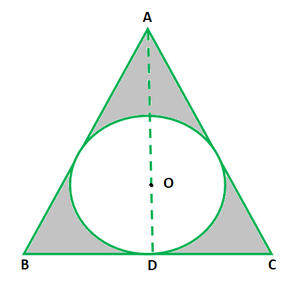Given that,

Side of ABC triangle = 12 cm

So, the area of the equilateral triangle = √3/4(side)2

= √3/4(12)2 = 36√3 cm2

Also the perimeter of triangle ABC = 3 x 12 = 36 cm

Now we find the radius of incircle = Area of triangle/ ½ (perimeter of triangle)

= 36√3/ 1/2 x 36

= 2√3 cm

Also, we find the area of the shaded part = Area of equilateral triangle – Area of circle

= 36√3 – πr2

= 36(1.732) – (3.14)(2√3)2

= 62.352 – 37.68

= 24.672 cm2

Hence, the area of the shaded part is 24.672 cm2

Question 25. In the figure, an equilateral triangle ABC of side 6 cm has been inscribed in a circle. Find the area of the shaded region. (Take π = 3.14)

Solution: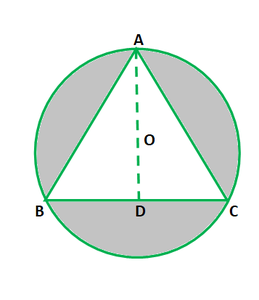Given that,

The side of ABC triangle = 6 cm

So, the area of the equilateral triangle = √3/4(side)2

= √3/4(6)2

= √3/4(36)

= 9√3 cm2

Let us mark the centre  of the circle as O, OA and OB are the radii of the circle.

In triangle BOD,

sin 60o = BD/ OB

√3/2 = 3/ OB

OB = 2√3 cm = r

Now we find the area of shaded region = Area of the circle – area of the equilateral triangle

= πr2 – 9√3

= 3.14 x (2√3)2 – 9√3

= 3.14 x 12 – 9 x 1.732

= 37.68 – 15.588

= 22.092 cm2

Hence, the area of the shaded region 22.092 cm2

Question 26. A circular field has a perimeter of 650 m. A square plot having its vertices on the circumference of the field is marked in the field. Calculate the area of the square plot.

Solution: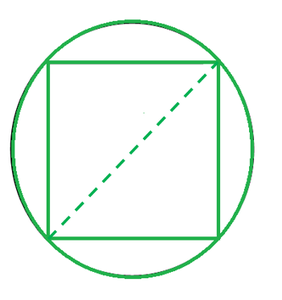Given that, the circular field has a perimeter = 650 m

As we know that the circumference of circle = 2πr

650 = 2×22/7xr

r = (650×7)/44

r = 103.409 m

As the diagonal of the square plot is the diameter of the circle.

Hence, r × 2 = d

So, diameter = 103.409 x 2

As we know that the Diameter of circle = 206.818 m = Diagonal of square plot

Now we find the area of the square plot = 1/2 × d²

= 1/2 × (206.818)²

= 1/2 × 42773.68

Hence, the area of the square plot is 21386.84 m2

Question 27. Find the area of a shaded region in the figure, where a circular arc of radius 7 cm has been drawn with vertex A of an equilateral triangle ABC of side 14 cm as centre. (use π = 22/7 and √3 = 1.73)

Solution: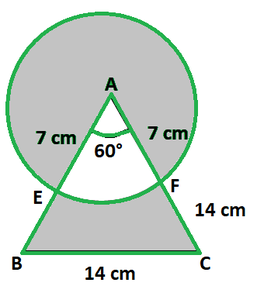Given that,

The radius of circle (r) = 7 cm.

Side of the triangle = 14 cm

Now we find the area of shaded region = Area of the circle + Area of the triangle – 2 area of the sector EAF

= πr2 + √3a2/4 – 2 × πr2 × 60/360

= 22/7 × (7)2 + (1.73)(14)2/4 – 2 × 22/7 × (7)2 × 60/360

= 283.77 – 51.33

= 187.43cm2

Hence, the area of the shaded region is 187.43cm2

Question 28. A regular hexagon is inscribed in a circle. If the area of hexagon is 24√3 cm2, find the area of the circle. (Use π = 3.14)

Solution: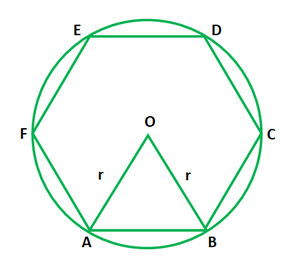Given that,

A regular hexagon ABCDEF is inscribed in a circle

Area of hexagon = 24 √3 cm2

Let us considered r be the radius of circle

So, the side of regular hexagon = r

Area of equilateral ΔOAB = √3/3 r2 cm2

But the area of triangle OAB = 1/6 x area of hexagon

24√3/6 = 4√3 r2

So, r2 = 4√3 x 4/√3 = 16

r = 4 cm

Now we find the area of circle = πr2 = 3.14 x (4)2 cm2

= 3.14 x 16 cm2

= 50.24 cm2

Hence, the area of circle = 50.24 cm2

(ii) the circle in which the hexagon is inscribed.

Solution: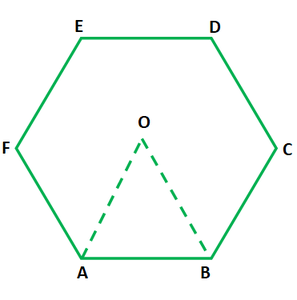The area of hexagon = 54 cm2

and area of circle = 65.324 cm2

Given that,

Area of ∆OAB = 9 cm2

Let us considered ‘r’ be the radius of a circle and ‘a’ be the side of a equilateral triangle.

(i) As we know that the area of hexagon = 6 × Area of equilateral triangle

= 6 × 9 = 54 cm²

= 54 cm²

(ii) Area of equilateral ∆OAB = √3/4 × a2

9 = √3/4 × a2

9 × 4 = √3a2

36 = √3a2

a2 = 36/√3

Side2 = 36/√3 cm

As we know that in regular hexagon inscribed in a circle,

its side is equal to the radius of a Circle

So, the radius of circle(r) = Side of a hexagon

r2 =  36/√3 cm

Now we find the area of circle = πr2

= 22/7 × 36/√3

= 22/7 × 36/1.732

= (22 × 36) /(7 ×1.732)

= 792/12.124

= 65.324 cm2

Hence, the area of hexagon is 54 cm2 and area of circle is 65.324 cm2

Question 30. Four equal circles, each of radius 5 cm, touch each other as shown in the figure. Find the area included between them. (Take π = 3.14).

Solution: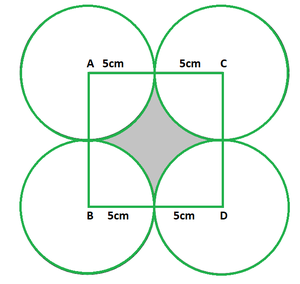Given that,

Radius of a circle = 5 cm

Side of a square = 2 × Radius of a circle

= 2 × 5

Side of a square = 10 cm

Area of a square = Side2

Area of a square = 102 = 100 cm2

Area of a square = 100 cm2

Area of the quadrant of one circle = 1/4πr2

Area of the quadrant of four circles = 4 × 1/4πr2 = πr2

= 3.14 × 5 × 5

= 3.14 × 25

= 78.5cm²

Area of the quadrant of four circles = 78.5cm2

Now we find the area of the shaded portion = Area of the square – Area of the quadrant of four circles

= 100 – 78.5

= 21.5 cm2

Hence, the area of the shaded portion is 21.5 cm2.

Question 31. Four equal circles, each of radius ‘a’ touch each other. Show that the area between them is 6a2/7. (Take π = 22/7)

Solution: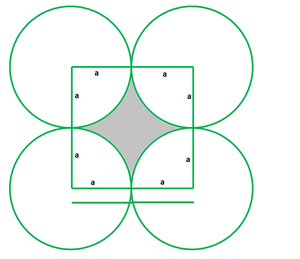Given that,

The radius of a circle = a

Side of a square = 2 × Radius of a circle

= 2 × a

Side of a square = 2a cm

Area of a square = Side2

= (2a)2

= 4a2

Area of the quadrant of one circle = 1/4πr2

Area of the quadrant of four circles = 4 × 1/4πr2 = πr2

Now we find the area of the shaded portion = Area of the square – Area of the quadrant of four circles

= 4a2 – 22/7 × a2

= 4a2 – 22a2/7

= (28a2 -22a2)/7

= 6a2/7

Hence, proved the area between them is 6a2/7

Question 32. A child makes a poster on a chart paper drawing a square ABCD of side 14 cm. She draws four circles with centre A, B, C, and D in which she suggests different ways to save energy. The circles are drawn in such a way that each circle touches externally two of the three remaining circles in the given figure. In the shaded region she write a message ‘Save Energy’. Find the perimeter and area of the shaded region. (Use π = 22/7)

Solution: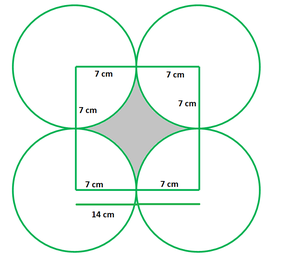Given that,

Side of a square = 14 cm

Radius of a Circle(r) = Side of a square/2 = 14/2 = 7 cm

Central angle, θ = 90°

So, the perimeter of the shaded portion = 4 × length of the arc having Central angle 90°

= 4 × θ/360° × 2πr

= 4 × 90°/360° × 2 × 22/7 × 7

= 4 × 1/4 × 44

= 44 cm

As we know that area of a square = Side2

= (14)2 = 196 cm2

Also, the area of a square = 196 cm2

Area of the quadrant of one circle = 1/4πr2

Area of the quadrant of four circles = 4 × 1/4πr2 = πr2

= 22/7 × 72

= 22 × 7

= 154 cm2

Now we find the area of the shaded portion = Area of the square ABCD – Area of the quadrant of four circles

= 196 – 154

= 42 cm2

Hence, the Perimeter of the shaded portion is 44 cm and area of the shaded portion is 42 cm2

Question 33. The diameter of a coin is 1 cm (see figure). If four such coins be placed on a table so that the rim of each touches that of the other two, find the area of the shaded region (Take π = 3.1416).

Solution: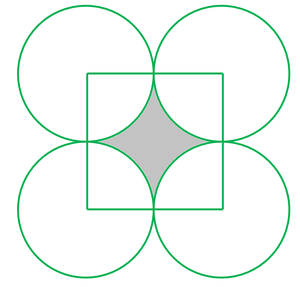Given that,

The diameter of a coin (circle) = 1 cm

Find: the area of the shaded region

As we know that the radius(r) of a coin (circle) = Diameter of a coin (circle)/2

r = 1/2 = 0.5 cm

Side of a square = 2 × Radius of a coin (circle)

= 2 × 0.5

= 1 cm

Area of the quadrant of one circle = 1/4πr2

Area of the quadrant of four circles = 4 × 1/4πr2 = πr2

Now we find the area of the shaded portion = Area of the square – Area of the quadrant of four circles

A = Side2 – πr2

= 12 – 3.1416 × (0.5)2

= 1 – 3.1416 × 0.25

= 1 – 0.7854

= 0.2146 cm2

Hence, the area of the shaded portion is 0.2146 cm2

Question 34. Two circular pieces of equal radii and maximum area, touching each other are cut out from a rectangular card board of dimensions 14 cm x 7 cm. Find the area of the remaining card board. (Use π = 22/7)

Solution: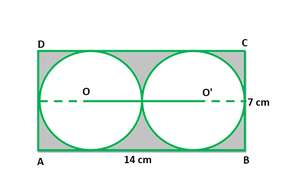Given that,

Length of a rectangle(l) = 14 cm

Breadth of a rectangle(b) = 7 cm

So, the diameter of 1 circle = 7 cm

Radius of 1 circle(r) = 7/2 cm

Now we find the area of the remaining cardboard = Area of rectangular cardboard – 2 × Area of circle

= l × b – 2 (πr2)

= (14× 7) – 2 × (22/7) × (7/2)2

= 98 – (44/7)(49/4)

= 98 – 77

= 21 cm2

Hence, the area of the remaining cardboard is 21 cm2.

My Personal Notes arrow_drop_up